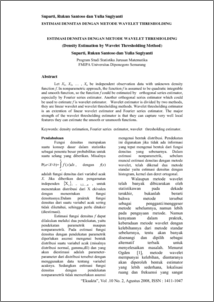# ESTIMASI DENSITAS DENGAN METODE WAVELET THRESHOLDING (Density Estimation by Wavelet Thresholding Method)

Suparti, Suparti and Santoso, Rukun and Sugiyanti, Yulia Sugiyanti (2008) ESTIMASI DENSITAS DENGAN METODE WAVELET THRESHOLDING (Density Estimation by Wavelet Thresholding Method). Jurnal EKSAKTA FMIPA UII Jogja, 10 (2). pp. 6-16. ISSN 1411-1047Preview
PDF
173Kb

Official URL: http:/lp2m.uii.ac.id

## Abstract

Let X1, X2, … , Xn be independent observation data with unknown density function f. In nonparametric approach, the function f is assumed to be quadratic integrable and smooth function, so the function f could be estimated by orthogonal series estimator, especially by Fourier series estimator. Another orthogonal series estimator which could be used to estimate f is wavelet estimator. Wavelet estimator is divided by two methods, they are linear wavelet and wavelet thresholding methods. Wavelet thresholding estimator is an extention of linear wavelet estimator and Fourier series estimator. The major strength of the wavelet thresholding estimator is that they can capture very well local features they can estimate the smooth or unsmooth functions. Keywords: density estimation, Fourier series estimator, wavelet thresholding estimator.

Item Type: Article Q Science > Q Science (General) Faculty of Science and Mathematics > Department of Statistics 3459 INVALID USER 13 Jan 2010 12:36 13 Jan 2010 12:38

Repository Staff Only: item control page